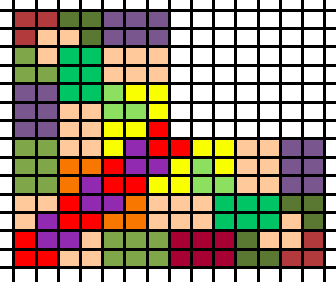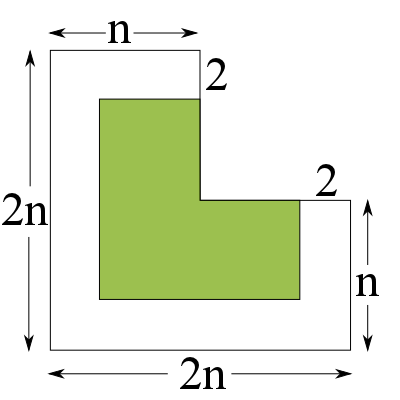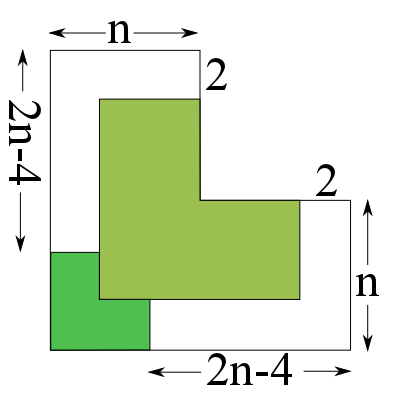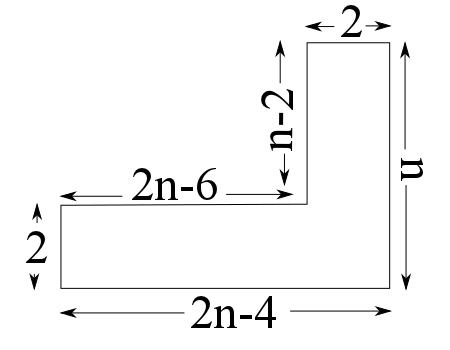#### You may also like### Doodles

Draw a 'doodle' - a closed intersecting curve drawn without taking pencil from paper. What can you prove about the intersections?### Russian Cubes

I want some cubes painted with three blue faces and three red faces. How many different cubes can be painted like that?### Picture Story

Can you see how this picture illustrates the formula for the sum of the first six cube numbers?

# L-triominoes

##### Age 14 to 16Challenge Level

Neerajan and Eilidh both noticed that the number of L-triominoes needed to tile a size $n$ L-triominoes was equal to $n^2$.

Philip from Wilson's School explained his thinking:

To tile a size 4 triomino you would simply put 4 size 2 triominoes together the same way you put four size 1 triominoes together to make a size 2.

Therefore you would put 4 size 4 triominoes together in the same way to form a size 8 triomino. Then 4 size 8 triominoes to make a size 16, and so on.

The triominoes could therefore tile size 8, 16, 32... $2^n$. These can therefore be made up of size 1 triominoes.

As it takes $4^1$ size 1 triominoes to tile a size 2 triomino, and $4^2$ size 1 triominoes to tile a size 4 triomino, it would therefore take $4^3=64$ size-1 triominoes to tile a size 8 triomino.
It would then take $4^4=256$ size 1 triominoes to tile a size 16 triomino and $4^5=1024$ size 1 triominoes to tile a size 32 triomino.
Finally, it would take $4^x$ triominoes to tile a size $2^x$ triomino.

To tile the size 3 triomino you simply use the 2x3 rectangular blocks at the top and on the right and then the rest you use a size 2 triomino and the size 1 in the middle.

To tile a size 5 triomino you simply add another size 2 triomino in the bottom left and for the rest you can use the 2x3 rectangular boxes.

Issac from Hampton School also managed to explain how to construct trionimoes of size $2^n$, and of size $3$ - well done Isaac!

Rajeev from Habedashers' Aske's Boys' School managed to find a way to colour a size-7 trionimo:The submitters above have managed to show that we can tile any L-trionimo of size $2^n$ by splitting it into four L-trionimoes of size $2^{n-1}$, all the way back to size-1 trionimoes. Now, let's think about how we can show that we can tile any L-trionimo of odd size:

Obviously, we can tile a size-1 trionimo with itself. Phillip, Isaac and Rajeev have shown above that it's possible to to tile trionimos of sizes three, five, and seven.

Suppose we have a trionimo of size $n$, where $n$ is an odd number, and we know that we can use L-trionimos to tile a trionimo of size $n-2$ (i.e. the next odd number down). Can we use this fact to help us tile our trionimo?

Let's try splitting our trionimo into a size $(n-2)$ trionimo and a surrounding L-shape:We are assuming we can tile the green area (the size $(n-2)$ trionimo). If we can find a way to tile the surrounding white area, then we can tile the whole shape and we are done.

Can we do this?

Yes! In fact, it is always possible (for any odd number $n$) to tile this area using only our two building blocks from before: the size-$2$ trionimo, and the $2 \times 3$ rectangle obtained by joining two trionimos together.

Let's try putting a size-$2$ trionimo in the bottom-left-hand corner of our shape:This leaves us with two white L-shapes to tile. We can see though, by the symmetry of the triomino, that these L-shapes are mirror images of each other, and so if we can find a way to tile one of them, we can easily use this to tile the other shape, and we are done. Let's focus on the bottom-right hand area:How can we tile this area using our building blocks? This time, the answer depends on $n$.
Every number $n$ is either a multiple of $3$, has remainder $1$ when divided by $3$, or has remainder $2$ when divided by $3$.

If $n$ is a multiple of $3$, the right-hand side of this shape (the vertical $2 \times n$ rectangle) can be covered completely with a line of $2 \times 3$ rectangles. This leaves a $(2n-6) \times 2$ rectangle untiled. However, if $n$ is a multiple of three, then so is $2n$, and as $6$ is a multiple of $3$ then so is $2n-6$. This means we can cover this remaining rectangle with another line of $2 \times 3$ rectangles, and we have successfully tiled the whole shape.

If $n$ has remainder $1$ when divided by $3$, we can fill in the bottom-right corner with a size-$2$ trionimo. This leaves us with two separate rectangles to fill in: a vertical one of size $2 \times (n-4)$, and a horizontal one of size $(2n-8) \times 2$.

As $n$ has remainder $1$ when divided by $3$, $n-1$ must be a multiple of $3$. Subtracting $3$ from this, we can see that $n-4$ is also a multiple of $3$ and so we can fill in our $2 \times (n-4)$ rectangle with a column of $2 \times 3$ rectangles.

Doubling a multiple of $3$ gives another multiple of $3$, so $2 \times (n-1) = 2n-2$ must also be a multiple of $3$. Subtracting $6$ (another multiple of $3$) from this, we can see that $2n-8$ is a multiple of $3$, so we can tile our $(2n-8) \times 2$ rectangle with a row of $3 \times 2$ rectangles, and we are done.

If $n$ has remainder $2$ when divided by $3$, $n-2$ must be a multiple of $3$. Doubling this, we can see that $2n-4$ must also be a multiple of three. This means that we can tile the bottom of this L-shape (the $(2n-4) \times 2$ rectangle) with a row of $3 \times 2$ rectangles, leaving a vertical rectangle of dimensions $2 \times (n-2)$.  However, we know that $n-2$ is also a multiple of three, so we can also fill in this rectangle with a column of $2 \times 3$ rectangles, and we are done.

So, regardless of what $n$ actually is, we can find some way to tile the white shape with trionimoes.

This now means that we can tile every trionimo of odd size!

Why?

We assumed that we already know how to tile a trionimo of size $n-2$. This means we now know that if we can tile a trionimo of size $n-2$, we can also tile a trionimo of size $n$ (as long as $n$ is odd).

However, Phillip, Isaac and Rajeev have shown how to tile a trionimo of size $3$.
Using what we have shown above, this means we can tile a trionimo of size $5$.
But if we can tile a trionimo of size $5$, we can tile a trionimo of size $7$!
And if we can tile a trionimo of size $7$, we can tile a size-$9$ triomino...

Carrying on like this, we can now see that we can tile any trionimo of odd size, no matter how big it is.

Can we combine everything we've done to show that we can, in fact, tile a triomino of any size?

Let's take a triomino of size $n$. If $n$ is odd, we know that we can tile it, so let's assume that $n$ is even.

Then, in exactly the same way that we tiled a size-$2^n$ triomino with four size-$2^{n-1}$ triominoes, we can use four triominoes of size $\frac{n}{2}$ to tile our trionimo.

How does this help?

Well, if we can tile each of these four triominoes, we can certainly tile the whole shape. So we know that if we can tile a trionimo of size $\frac{n}{2}$, we can tile a trionimo of size $n$.

If $\frac{n}{2}$ is odd, we know that we can tile it, and we're done.

If $\frac{n}{2}$ is even, we can repeat the entire process: tile a $\frac{n}{2}$-size trionimo with four size-$\frac{n}{4}$ trionimoes and we can see that if we can tile a size-$\frac{n}{4}$ triomino, we can tile a size-$n$ triomino.

If $\frac{n}{4}$ is odd, then we're finished, and if it's even we repeat the process again.

No matter how many times we have to repeat this process, eventually we'll stop at an odd number.
(Can you see why this is?)

So eventually, we will be able to find an odd number $m$ such that we can tile our trionimo with size-$m$ triominoes.

As $m$ is odd, we know that we can replace each size-$m$ trionimo with a tiling of $m^2$ single triominoes, and we have found a way to tile our size-$n$ triomino with single L-triominoes.

This works for any even number $n$.

So we can tile any odd-sized triomino and any even-sized triomino. Putting this together, we can tile any triomino using size-$1$ triominoes!